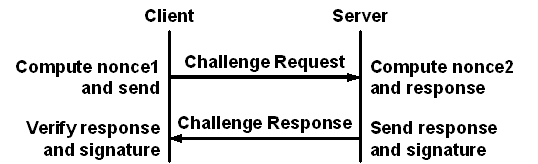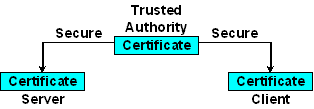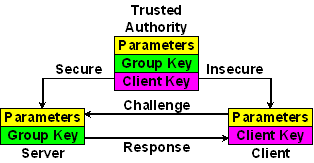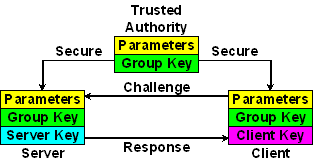# Autokey Identity SchemesMaya glyph alautun

#### Introduction

While the identity scheme described in RFC-2875 is based on a ubiquitous Diffie-Hellman infrastructure, it is expensive to generate and use when compared to others described here. There are five schemes now implemented in Autokey to prove identity: (1) trusted certificates (TC), (2) private certificates (PC), (3) a modified Schnorr algorithm (IFF aka Identify Friendly or Foe), (4) a modified Guillou-Quisquater algorithm (GQ), and (5) a modified Mu-Varadharajan algorithm (MV). The TC scheme, which involves a certificate trail to a trusted host, is discussed on the Autokey Protocol page. Each of the others involves generating parameters specific to the scheme, together with public and private values used by the scheme.

In order to simplify implementation, each scheme uses existing structures in the OpenSSL library, including those for RSA and DSA cryptography. As these structures are sometimes used in ways far different than their original purpose, they are called cuckoo structures in the descriptions that follow.In the challenge-response schemes client Alice asks server Bob to prove identity relative to a secret group key b provided by a trusted authority (TA). As shown in the figure above, client Alice rolls random nonce1 and sends to server Bob. Bob rolls random nonce2, performs some mathematical function and returns the response along with the hash of some private value to Alice. Alice performs another mathematical function and verifies the result matches the hash in Bob's message.

Each of the five schemes is intended for specific use. There are three kinds of keys, trusted agent, server and client. Servers can be clients of other servers, but clients cannot cannot be servers for dependent clients. In general, the goals of the schemes are that clients cannot masquerade as a servers and a servers cannot masquerade as a trusted agents (TAs), but they differ somewhat on how to achieve these goals. To the extent that identity can be verified without revealing the group key, the schemes are properly described as zero-knowledge proofs.

The four identity schemes described here have different design goals and are intended for specific application. The PC scheme is intended for one-way broadcast configurations where clients cannot run a duplex protocol. It is essentially a symmetric key cryptosystem where the certificate itself is the key.

The IFF scheme is intended for servers operated by national laboratories. The servers share a private group key and provide the public client parameters on request. The clients cannot masquerade as servers.

The GQ scheme is intended for exceptionally hostile scenarios where it is necessary to change the client key at relatively frequent intervals. As in the IFF scheme the servers share a private group key and provide the public client parameters on request. In this scheme clients requre a public key to complete the exchange. This is conveyed in the server certificate in an extension field. The certificate can be changed while retaining the same group key.

The MV scheme is intended for the most challenging scenarios where it is neccesary to protect against both server and client masquerade. The private values used by the TA to generate the cryptosystem are not available to the servers and the private values used by the servers to encrypt data are not available to the clients. Thus, a client cannot masquerade as a server and a server cannot masquerade as a TA. However, a client can verify a server has the correct group key even though neither the client nor server know the group key, nor can either manufacture a client key acceptable to any other client. A further feature of this scheme is that the TA can collaborate with the servers to revoke client keys.

#### Private Certificate (PC) CryptosystemThe PC scheme shown above uses a private certificate as the group key. A certificate is designated private by a X509 Version 3 extension field when generated by the ntp-keygen program in the NTP software distribution. In the Autokey context it functions as a symmetric key. The private certificate is generated by a TA and distributed to all group members by secure means and is never revealed outside the group. A client is marked trusted in the (optional) Parameter Exchange and authentic when the first signature is verified. This scheme is cryptographically strong as long as the private certificate is protected; however, it can be very awkward to refresh the keys or certificate, since new values must be securely distributed to a possibly large population and activated simultaneously.

#### Schnorr (IFF) Cryptosystem

The Schnorr (IFF) identity scheme can be used when certificates are generated by utilities other than the ntp-keygen program in the NTP software distribution. Certificates can be generated by the OpenSSL library or an external public certificate authority, but conveying an arbitrary public value in a certificate extension field might not be possible. The TA generates the IFF parameters, private key and public key, then sends these values to the servers and the parameters and public key to the clients. Without the private key a client cannot masquerade as a legitimate server.

The DSA parameters are generated by routines in the OpenSSL library. The IFF values hide in a DSA cuckoo structure which uses the same parameters. The values are used by an identity scheme based on DSA cryptography and described in  and  p. 285. The p is a 512-bit prime, g a generator of the multiplicative group Zp* and q a 160-bit prime that divides p - 1 and is a qth root of 1 mod p; that is, gq = 1 mod p. The TA rolls a private random group key b (0 < b < q) and computes the public key v = gb, then distributes private (p, q, g, b) to the servers using secure means and public (p, q, g, v) to the clients not necessarily using secure means.The TA generates a DSA parameter structure for use as IFF parameters. The IFF parameters are identical to the DSA parameters, so the OpenSSL library DSA parameter generation routine can be used directly. The DSA parameter structure is written to a file as an encrypted DSA key encoded in PEM. Unused structure members are set to one.

 IFF DSA Item Include p p modulus all q q modulus all g g generator all b priv_key group key server v pub_key client key client

Alice challenges Bob to confirm identity using the following protocol exchange.

1. Alice rolls random r (0 < r < q) and sends to Bob.
2. Bob rolls random k (0 < k < q), computes y = k + b r mod q and x = gk mod p, then sends (y, hash(x)) to Alice.
3. Alice computes z = gy vr mod p and verifies hash(z) equals hash(x).

#### Guillou-Quisquater (GQ) Cryptosystem

The Guillou-Quisquater (GQ) identity scheme is useful when certificates are generated by the ntp-keygen program in the NTP software distribution. The TA generates the GQ parameters, private key and public key, then sends these values to the servers and the parameters to the clients. The public key is inserted in an X.509 extension field when the certificate is generated. Without the private key a client cannot masquerade as a legitimate server.

The RSA parameters are generated by routines in the OpenSSL libbrary. The GQ values hide in a RSA cuckoo structure which uses the same parameters. The values are used in an identity scheme based on RSA cryptography and described in  and  p. 300 (with errors). The 512-bit public modulus n = p q, where p and q are secret large primes. The TA rolls random group key b (0 < b < n) and sends (n, b) to the servers using secure means. The private key and public key are constructed later.The TA generates a RSA parameter structure for use as GQ parameters. The RSA parameter structure is written to a file as an encrypted RSA key encoded in PEM. Unused structure members are set to one.

When generating new certificates, the server rolls new random private key u (0 < u < n) and public key its inverse u-1 obscured by the group key v = u-1 b. These values replace the private and public keys normally generated by the RSA scheme. In addition, the public key v is conveyed in a X.509 certificate extension.

 GQ RSA Item Include n n modulus all b e group key all u p server key server v q client key client

Alice challenges Bob to confirm identity using the following exchange.

1. Alice rolls random r (0 < r < n) and sends to Bob.
2. Bob rolls random k (1 < k < n) and computes y = k ur mod n and x = kb mod n, then sends (y, hash(x)) to Alice.
3. Alice computes z = vr yb mod n and verifies hash(z) equals hash(x).

#### Mu-Varadharajan (MV) Cryptosystem

The Mu-Varadharajan (MV) scheme was originally intended to encrypt broadcast transmissiions to receivers which do not transmit. There is one encryption key for the broadcaster and a separate decryption key for each receiver. It operates something like a pay-per-view satellite broadcasting system where the session key is encrypted by the broadcaster and the decryption keys are held in a tamperproof set-top box. We don't use it this way, but read on.

In the MV scheme the TA constructs an intricate cryptosystem involving a number of activation keys known only to the TA. The TA decides which keys to activate and provides to the servers an encryption key E and server decryption keys gbar and ghat which depend on the activated keys. The servers have no additional information and, in particular, cannot masquerade as a TA. In addition, the TA provides for each activation key j individual client decryption keys xbar and xhat, which do not need to be changed if the TA enables or disables an activation key. The clients have no further information and, in particular, cannot masquerade as a server or TA.

Clients are assigned one of the activation keys and are provided with the corresponding client key. There can be any number of clients sharing the same activation key according to policy. While the machinery to enable and disable ativation keys is included in the current implementation, specific means and interfaces to do this are not yet available, so only one client key is provided.

The scheme is designed so that clients can construct the inverse of E from the server gbar and ghat and client xbar and xhat. In the scheme both E and its inverse are exponentiated by a server nonce, so the product is always one and the secrecy depends on the descrete log problem.

The MV values hide in a DSA cuckoo structure which uses the same parameters, but generated in a different way. The values are used in an encryption scheme similar to El Gamal cryptography and use a polynomial formed from the expansion of product terms (x - xj), as described in . The paper has significant errors and serious omissions.The TA generates the modulus, encryption key and server decryption keys as an encrypted DSA key encoded in PEM. Unused structure members are set to one.

 MV DSA Item Include p p modulus all q q modulus server E g private encrypt key server gbar priv_key server decrypt key server ghat pub_key server decrypt key server

The TA generates the modulus and client decryption keys as a nonencrypted DSA key encoded in PEM. It is used only by designated recipient(s) who pay a suitably outrageous fee for its use. Unused structure members are set to one.

 MV DSA Item Include p p modulus all xbar priv_key client decrypt key client xhat pub_key client decrypt key client

The devil is in the details. Let q be the product of n distinct primes s1j (j = 1...n), where each s1j, also called an activation key, has m significant bits. Let prime p = 2q + 1, so that q and each s1j divide p - 1 and p has M = nm + 1 significant bits. Let g be a generator of the multiplicative group Zp*; that is, gcd(g, p - 1) = 1 and gq = 1 mod p. We do modular arithmetic over Zq and then project into Zp* as powers of g. Sometimes we have to compute an inverse b-1 of random b in Zq, but for that purpose we require gcd(b, q) = 1. We expect M to be in the 500-bit range and n relatively small, like 30. The TA uses a nasty probabilistic algorithm to generate the cryptosystem. In the following let the number of bits in the modulus m = 512.

1. The object is to generate a multiplicative group Zp* modulo a prime p and a subset Zq mod q, where q is the product of n distinct m-bit primes s1j (j = 1...n) and q divides p - 1. As a practical matter, it is tough to find more than 31 distinct primes for mn = 512 or 61 primes for mn = 1024. The latter can take several hundred iterations and several minutes on a Sun Blade 1000.
2. Compute the modulus q as the product of the primes. Compute the modulus p as 2q + 1 and test p for primality. If p is composite, replace one of the primes with a new distinct prime and try again. Note that q will hardly be a secret since we have to reveal p to servers and clients. However, factoring q to find the primes should be adequately hard, as this is the same problem considered hard in RSA. Question: is it as hard to find n small prime factors totalling n bits as it is to find two large prime factors totalling n bits? Remember, the bad guy doesn't know n.
3. Associate with each s1j an element sj such that sj s1j = s1j mod q. One way to find an sj is to compute the quotient (q + s1j) / s1j mod p. The student should prove the remainder is always zero.
4. Compute the generator g of Zp using a random roll such that gcd(g, p - 1) = 1 and gq = 1. If not, roll again.

The cryptosystem parameters n, p, q, g, s1j, sj (j = 1...n) have been determined. The TA sets up a specific instance of the scheme as follows.

1. Roll random roots xj mod q (j = 1...n) for a polynomial of order n. While it may not be strictly necessary, Make sure each root has no factors in common with q.
2. Generate polynomial coefficients ai (i = 0...n) from the expansion of root products (x - xi) mod q in powers of x using a fast method contributed by Charlie Boncelet.
3. Generate gi = gai mod p for all i and the generator g. Verify prod(giai xji) = 1 for all i, j. Note the ai xji exponent is computed mod q, but the gi is computed mod p. Also note the expression given in the paper cited is incorrect.
4. Make master encryption key A = Prod(gixj) (i = 0...n, j = 1...n - 1). Keep it around for awhile, since it is expensive to compute.
5. Roll private random group key b mod q (0 < b < q), where gcd(b, q) = 1 to guarantee the inverse exists, then compute b-1 mod q. If b is changed, all keys must be recomputed.
6. Make private client keys xbarj = b-1 Sum(xin mod q) (i = 1...n, i != j) and xhatj = sjxjn for all j. Note that the keys for the jth client involve only sj and that s1j remain secret. The TA sends (p, xbarj, xhatj) to the jth client(s) using nonsecure means.
7. The activation key is initially q by construction. The TA revokes client j by dividing q by s1j. The quotient becomes the activation key s. Note we always have to revoke one key; otherwise, the plaintext and cryptotext would be identical. The TA computes private server encryption key E = As and server decryption keys gbar = gbars and ghat = ghatsb mod p and sends (p, E, gbar, ghat) to the servers using secure means. These values must be recomputed if an activation key is changed.

Alice challenges Bob to confirm identity using the following exchange.

1. Alice rolls random r (0 < r < q) and sends to Bob.
2. Bob rolls random k (0 < k < q), computes y = rEk, ybar = gbark and yhat = ghatk, then returns (y, ybar, yhat) to Alice.
3. Alice computes the session decryption key (Ek)-1 = ybarxhatj yhatxbarj, then verifies that y = r.

#### Selected Publications

1. Guillou, L.C., and J.-J. Quisquatar. A "paradoxical" identity-based signature scheme resulting from zero-knowledge. Proc. CRYPTO 88 Advanced in Cryptology, Springer-Verlag, 1990, 216-231.
2. Mills, D.L. The Autokey security architecture, protocol and algorithms. Electrical and Computer Engineering Technical Report 06-1-1, University of Delaware, January 2006, 59 pp, PDF.
3. Mu, Y., and V. Varadharajan. Robust and secure broadcasting. Proc. INDOCRYPT 2001, LNCS 2247, Springer Verlag, 2001, 223-231.
4. Schnorr, C.P. Efficient signature generation for smart cards. J. Cryptology 4, 3 (1991), 161-174.
5. Stinson, D.R. Cryptography - Theory and Practice. CRC Press, Boca Raton, FA, 1995, ISBN 0-8493-8521-0.